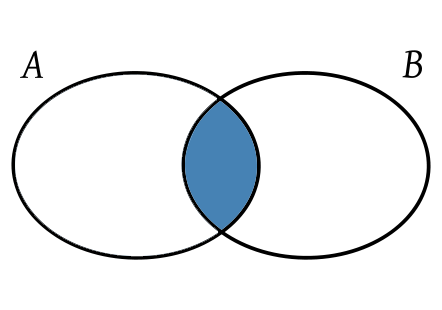# How to Get Array Intersection in JavaScript

JavaScript arrays are popular data structures used by web developers to easily store and manipulate data. Sometimes you may need to get array intersection in JavaScript, to obtain elements common to both arrays. In this article, we will learn how to get array intersection in JavaScript.

## How to Get Array Intersection in JavaScript

There are several ways to get array intersection in JavaScript. We will look at them one by one. Let us say you have the following 2 arrays.

```var arr1 = [1, 2, 3, 4];
var arr2 = [2, 3, 4, 5]```

We need to obtain the intersection of the above arrays as shown.

`[2,3,4]`

## 1. Using filter

Every JavaScript array has a built in function called filter() that allows you to iterate through the array items and perform custom operations while retaining the result of each iteration, so that you can use them in subsequent iterations. A simple filter to obtain intersection of 2 arrays is.

```arr3 = arr1.filter(elem => arr2.includes(elem));
console.log(arr3); //[2,3,4]```

The filter() function above will iterate through items of arr1, and check if each of them is present in arr2, using includes() function, which is also available out-of-the-box for each array. If an item in arr1 array is present in arr2 array, it is stored in the result array. Finally, when filter() function has completed iterating through arr1 array, it will return the result array.

You can include the above command in a JavaScript function if you want.

```function intersection(arr1, arr2) {
return arr1.filter(elem => arr2.includes(elem));
}```

## 2. Using Sets

In this case, we use Set function to compute the intersection of two arrays.

```function intersection(arr1, arr2) {
var set = new Set(arr2);
var intersection = new Set(arr1.filter(elem => set.has(elem)));
return Array.from(intersection);
}```

Let us look at the above function in detail. First, we create a set out of array arr2 using Set() constructor. Then we use it to get intersection set. In the constructor of intersection set, we use filter() function to specify that items in array arr1 are to be included only if they are also present in array arr2.

Finally, we use Array.from() function to construct an array from intersection set, and return it. In some cases, this approach might be faster, since we use has() function instead of using includes() function. has() function has a time complexity of O(1) while includes() function has a time complexity of O(n). But we need to convert arrays into sets and vice versa so that takes extra time.

In this article, we have learnt couple of ways to find intersection of two arrays in JavaScript. Calculating array intersections are useful if you want to find out which elements of your array also belong to another array.